# "Importing textdata from csv files"

Member, University Professor Posts: 6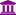University Professor
edited May 2019 in Help
I teach a data mining course to business management students (with little or no programming experience) using a combination of R and RapidMiner. I try to duplicate the examples from each package in the other so that students to appreciate the differences in usability, available algorithms and results. For obvious reasons I use the graphical process approach in RapidMiner, rather than teaching XML (which I don't know anyway).

I have two csv data sets which I use in R, neither of which I have been able to import in the appropriate format to use in RapidMiner, despite playing around with what look like sensible operator options.

sms_spam.csv has two columns, the first identifies the content of the second as "spam" or "ham", while the second is a text message. (http://www.dt.fee.unicamp.br/~tiago/smsspamcollection/). I want to import this so that I can use Naive Bayes to build a classifier for messages.

groceries.csv is an example set that comes with the arules library in R. It has multiple (unlabelled) columns, with each row representing a transaction, and as many columns used as there are items - so unstructured. I want to use association rules and/or fp-growth on this.

Any suggestions on how I can get either or both of these data sets into RapidMiner in a usable form would be greatly appreciated.

Tagged:

• Administrator, Moderator, Employee, RapidMiner Certified Analyst, Community Manager, Member, University Professor, PM Moderator Posts: 2,959Community Manager
hi @User33136 - good to hear from you. I took a minute on your sms-spam.csv set. Is this what you're looking for?

```<?xml version="1.0" encoding="UTF-8"?><process version="9.1.000">
<context>
<input/>
<output/>
<macros/>
</context>
<operator activated="true" class="process" compatibility="9.1.000" expanded="true" name="Process">
<parameter key="logverbosity" value="init"/>
<parameter key="random_seed" value="2001"/>
<parameter key="send_mail" value="never"/>
<parameter key="process_duration_for_mail" value="30"/>
<parameter key="encoding" value="SYSTEM"/>
<process expanded="true">
<operator activated="true" breakpoints="after" class="read_csv" compatibility="9.1.000" expanded="true" height="68" name="Read CSV" width="90" x="45" y="340">
<parameter key="csv_file" value="/Users/genzerconsulting/Desktop/sms_spam.csv"/>
<parameter key="column_separators" value=","/>
<parameter key="trim_lines" value="false"/>
<parameter key="use_quotes" value="true"/>
<parameter key="quotes_character" value="&quot;"/>
<parameter key="escape_character" value="\"/>
<parameter key="comment_characters" value="#"/>
<parameter key="starting_row" value="1"/>
<parameter key="parse_numbers" value="true"/>
<parameter key="decimal_character" value="."/>
<parameter key="grouped_digits" value="false"/>
<parameter key="grouping_character" value=","/>
<parameter key="infinity_representation" value=""/>
<parameter key="date_format" value=""/>
<parameter key="first_row_as_names" value="true"/>
<list key="annotations"/>
<parameter key="time_zone" value="SYSTEM"/>
<parameter key="locale" value="English (United States)"/>
<parameter key="encoding" value="UTF-8"/>
<list key="data_set_meta_data_information">
<parameter key="0" value="type.true.binominal.label"/>
<parameter key="1" value="text.true.text.attribute"/>
</list>
<parameter key="datamanagement" value="double_array"/>
<parameter key="data_management" value="auto"/>
</operator>
<operator activated="true" class="text:process_document_from_data" compatibility="8.1.000" expanded="true" height="82" name="Process Documents from Data" width="90" x="179" y="340">
<parameter key="create_word_vector" value="true"/>
<parameter key="vector_creation" value="TF-IDF"/>
<parameter key="keep_text" value="false"/>
<parameter key="prune_method" value="percentual"/>
<parameter key="prune_below_percent" value="1.0"/>
<parameter key="prune_above_percent" value="30.0"/>
<parameter key="prune_below_rank" value="0.05"/>
<parameter key="prune_above_rank" value="0.95"/>
<parameter key="datamanagement" value="double_sparse_array"/>
<parameter key="data_management" value="auto"/>
<parameter key="select_attributes_and_weights" value="false"/>
<list key="specify_weights"/>
<process expanded="true">
<operator activated="true" class="text:transform_cases" compatibility="8.1.000" expanded="true" height="68" name="Transform Cases" width="90" x="45" y="34">
<parameter key="transform_to" value="lower case"/>
</operator>
<operator activated="true" class="text:tokenize" compatibility="8.1.000" expanded="true" height="68" name="Tokenize" width="90" x="179" y="34">
<parameter key="mode" value="non letters"/>
<parameter key="characters" value=".:"/>
<parameter key="language" value="English"/>
<parameter key="max_token_length" value="3"/>
</operator>
<operator activated="true" class="text:filter_stopwords_english" compatibility="8.1.000" expanded="true" height="68" name="Filter Stopwords (English)" width="90" x="313" y="34"/>
<operator activated="true" class="text:filter_by_length" compatibility="8.1.000" expanded="true" height="68" name="Filter Tokens (by Length)" width="90" x="447" y="34">
<parameter key="min_chars" value="4"/>
<parameter key="max_chars" value="25"/>
</operator>
<connect from_port="document" to_op="Transform Cases" to_port="document"/>
<connect from_op="Transform Cases" from_port="document" to_op="Tokenize" to_port="document"/>
<connect from_op="Tokenize" from_port="document" to_op="Filter Stopwords (English)" to_port="document"/>
<connect from_op="Filter Stopwords (English)" from_port="document" to_op="Filter Tokens (by Length)" to_port="document"/>
<connect from_op="Filter Tokens (by Length)" from_port="document" to_port="document 1"/>
<portSpacing port="source_document" spacing="0"/>
<portSpacing port="sink_document 1" spacing="0"/>
<portSpacing port="sink_document 2" spacing="0"/>
</process>
</operator>
<operator activated="true" class="split_data" compatibility="9.1.000" expanded="true" height="103" name="Split Data" width="90" x="313" y="340">
<enumeration key="partitions">
<parameter key="ratio" value="0.8"/>
<parameter key="ratio" value="0.2"/>
</enumeration>
<parameter key="sampling_type" value="automatic"/>
<parameter key="use_local_random_seed" value="false"/>
<parameter key="local_random_seed" value="1992"/>
</operator>
<operator activated="true" class="concurrency:cross_validation" compatibility="9.1.000" expanded="true" height="145" name="Cross Validation" width="90" x="514" y="136">
<parameter key="split_on_batch_attribute" value="false"/>
<parameter key="leave_one_out" value="false"/>
<parameter key="number_of_folds" value="10"/>
<parameter key="sampling_type" value="automatic"/>
<parameter key="use_local_random_seed" value="false"/>
<parameter key="local_random_seed" value="1992"/>
<parameter key="enable_parallel_execution" value="true"/>
<process expanded="true">
<operator activated="true" class="naive_bayes" compatibility="9.1.000" expanded="true" height="82" name="Naive Bayes" width="90" x="112" y="34">
<parameter key="laplace_correction" value="true"/>
</operator>
<connect from_port="training set" to_op="Naive Bayes" to_port="training set"/>
<connect from_op="Naive Bayes" from_port="model" to_port="model"/>
<portSpacing port="source_training set" spacing="0"/>
<portSpacing port="sink_model" spacing="0"/>
<portSpacing port="sink_through 1" spacing="0"/>
</process>
<process expanded="true">
<operator activated="true" class="apply_model" compatibility="9.1.000" expanded="true" height="82" name="Apply Model" width="90" x="45" y="34">
<list key="application_parameters"/>
<parameter key="create_view" value="false"/>
</operator>
<operator activated="true" class="performance_binominal_classification" compatibility="9.1.000" expanded="true" height="82" name="Performance" width="90" x="179" y="34">
<parameter key="main_criterion" value="first"/>
<parameter key="accuracy" value="true"/>
<parameter key="classification_error" value="false"/>
<parameter key="kappa" value="false"/>
<parameter key="AUC (optimistic)" value="false"/>
<parameter key="AUC" value="false"/>
<parameter key="AUC (pessimistic)" value="false"/>
<parameter key="precision" value="false"/>
<parameter key="recall" value="false"/>
<parameter key="lift" value="false"/>
<parameter key="fallout" value="false"/>
<parameter key="f_measure" value="false"/>
<parameter key="false_positive" value="false"/>
<parameter key="false_negative" value="false"/>
<parameter key="true_positive" value="false"/>
<parameter key="true_negative" value="false"/>
<parameter key="sensitivity" value="false"/>
<parameter key="specificity" value="false"/>
<parameter key="youden" value="false"/>
<parameter key="positive_predictive_value" value="false"/>
<parameter key="negative_predictive_value" value="false"/>
<parameter key="psep" value="false"/>
<parameter key="skip_undefined_labels" value="true"/>
<parameter key="use_example_weights" value="true"/>
</operator>
<connect from_port="model" to_op="Apply Model" to_port="model"/>
<connect from_port="test set" to_op="Apply Model" to_port="unlabelled data"/>
<connect from_op="Apply Model" from_port="labelled data" to_op="Performance" to_port="labelled data"/>
<connect from_op="Performance" from_port="performance" to_port="performance 1"/>
<portSpacing port="source_model" spacing="0"/>
<portSpacing port="source_test set" spacing="0"/>
<portSpacing port="source_through 1" spacing="0"/>
<portSpacing port="sink_test set results" spacing="0"/>
<portSpacing port="sink_performance 1" spacing="0"/>
<portSpacing port="sink_performance 2" spacing="0"/>
</process>
</operator>
<operator activated="true" class="apply_model" compatibility="9.1.000" expanded="true" height="82" name="Apply Model (2)" width="90" x="648" y="340">
<list key="application_parameters"/>
<parameter key="create_view" value="false"/>
</operator>
<operator activated="true" class="performance_binominal_classification" compatibility="9.1.000" expanded="true" height="82" name="Performance (2)" width="90" x="782" y="340">
<parameter key="main_criterion" value="first"/>
<parameter key="accuracy" value="true"/>
<parameter key="classification_error" value="false"/>
<parameter key="kappa" value="false"/>
<parameter key="AUC (optimistic)" value="false"/>
<parameter key="AUC" value="false"/>
<parameter key="AUC (pessimistic)" value="false"/>
<parameter key="precision" value="false"/>
<parameter key="recall" value="false"/>
<parameter key="lift" value="false"/>
<parameter key="fallout" value="false"/>
<parameter key="f_measure" value="false"/>
<parameter key="false_positive" value="false"/>
<parameter key="false_negative" value="false"/>
<parameter key="true_positive" value="false"/>
<parameter key="true_negative" value="false"/>
<parameter key="sensitivity" value="false"/>
<parameter key="specificity" value="false"/>
<parameter key="youden" value="false"/>
<parameter key="positive_predictive_value" value="false"/>
<parameter key="negative_predictive_value" value="false"/>
<parameter key="psep" value="false"/>
<parameter key="skip_undefined_labels" value="true"/>
<parameter key="use_example_weights" value="true"/>
</operator>
<connect from_op="Read CSV" from_port="output" to_op="Process Documents from Data" to_port="example set"/>
<connect from_op="Process Documents from Data" from_port="example set" to_op="Split Data" to_port="example set"/>
<connect from_op="Split Data" from_port="partition 1" to_op="Cross Validation" to_port="example set"/>
<connect from_op="Split Data" from_port="partition 2" to_op="Apply Model (2)" to_port="unlabelled data"/>
<connect from_op="Cross Validation" from_port="model" to_op="Apply Model (2)" to_port="model"/>
<connect from_op="Apply Model (2)" from_port="labelled data" to_op="Performance (2)" to_port="labelled data"/>
<connect from_op="Performance (2)" from_port="performance" to_port="result 1"/>
<connect from_op="Performance (2)" from_port="example set" to_port="result 2"/>
<portSpacing port="source_input 1" spacing="0"/>
<portSpacing port="sink_result 1" spacing="336"/>
<portSpacing port="sink_result 2" spacing="0"/>
<portSpacing port="sink_result 3" spacing="0"/>
</process>
</operator>
</process>
```

I will give the other a try later.

Do you want me to put these on the community samples repo for your students? It may be easier for you and your students.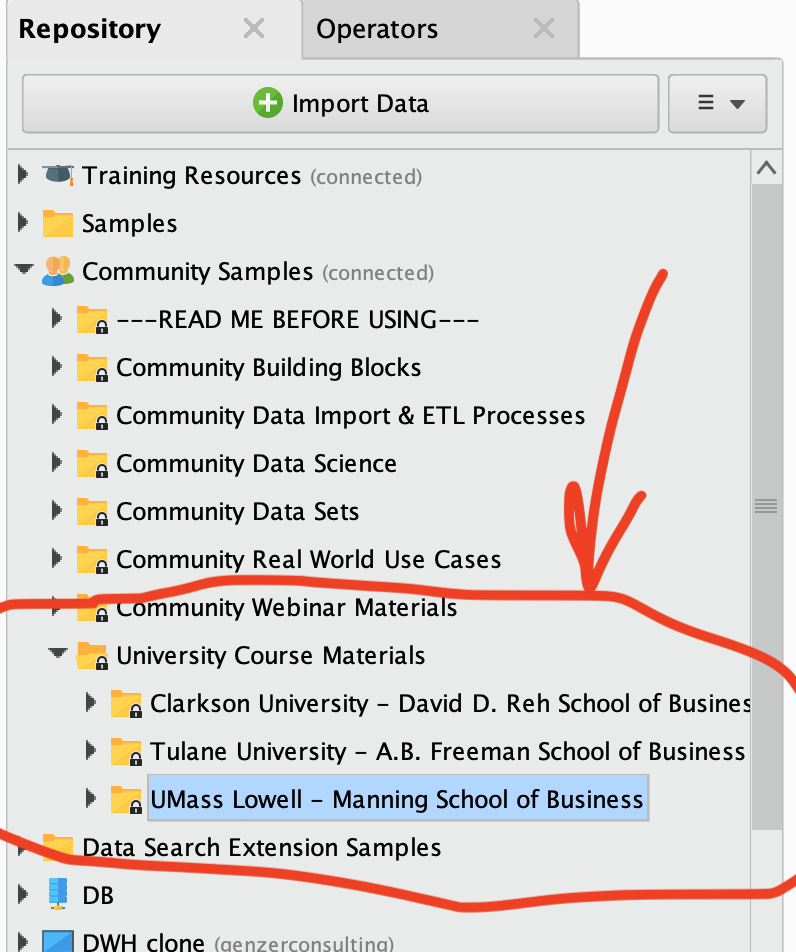Scott

• Member, University Professor Posts: 6University Professor

To be honest, I wouldn't know where to start with the code. I only ever use the GUI, and my students freak at the very thought of having to adapt the R code I give them.

I was hoping there were suitable operators available to do this - there are a few that sound like they should, but I can't get any of them to work.

Gary
(I would change my user name to my actual name, but apparently I lack the privileges to do this)
• Administrator, Moderator, Employee, RapidMiner Certified Analyst, Community Manager, Member, University Professor, PM Moderator Posts: 2,959Community Manager
Hi Gary -

Haha no only the community manager can change the user id.I can change this to whatever you want. Let me know what your preferred 'handle' is.

That code is XML - the backbone of RapidMiner and the way people swap processes. You can learn how to do it here: https://community.rapidminer.com/discussion/37047. It's just a matter of 'copy-and-paste'. I'm attaching the same process as an .rmp file to this message if that's easier for you.

The process looks like this when you load it into RapidMiner: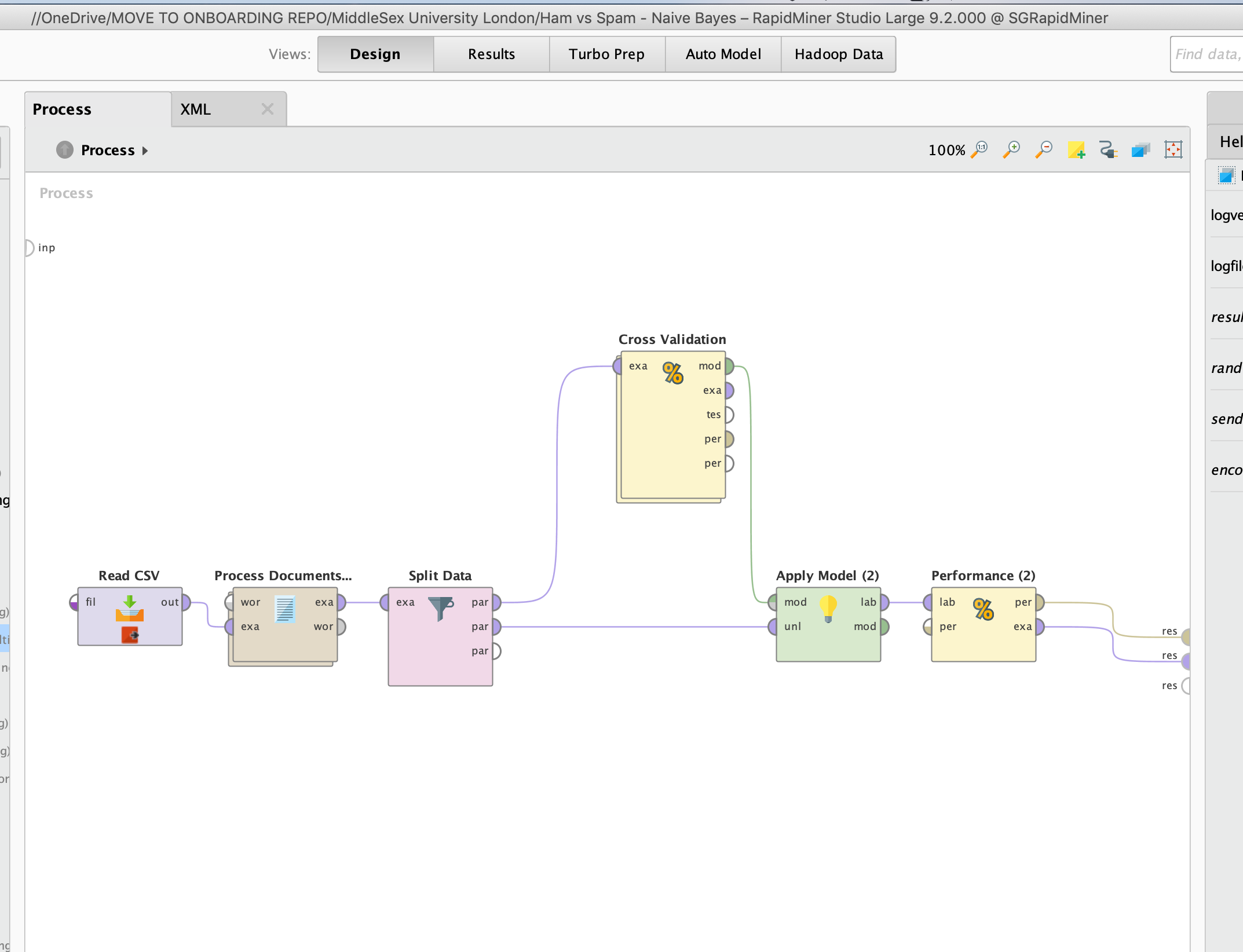and I made a folder for you on the Community Samples repo so any student can just load and run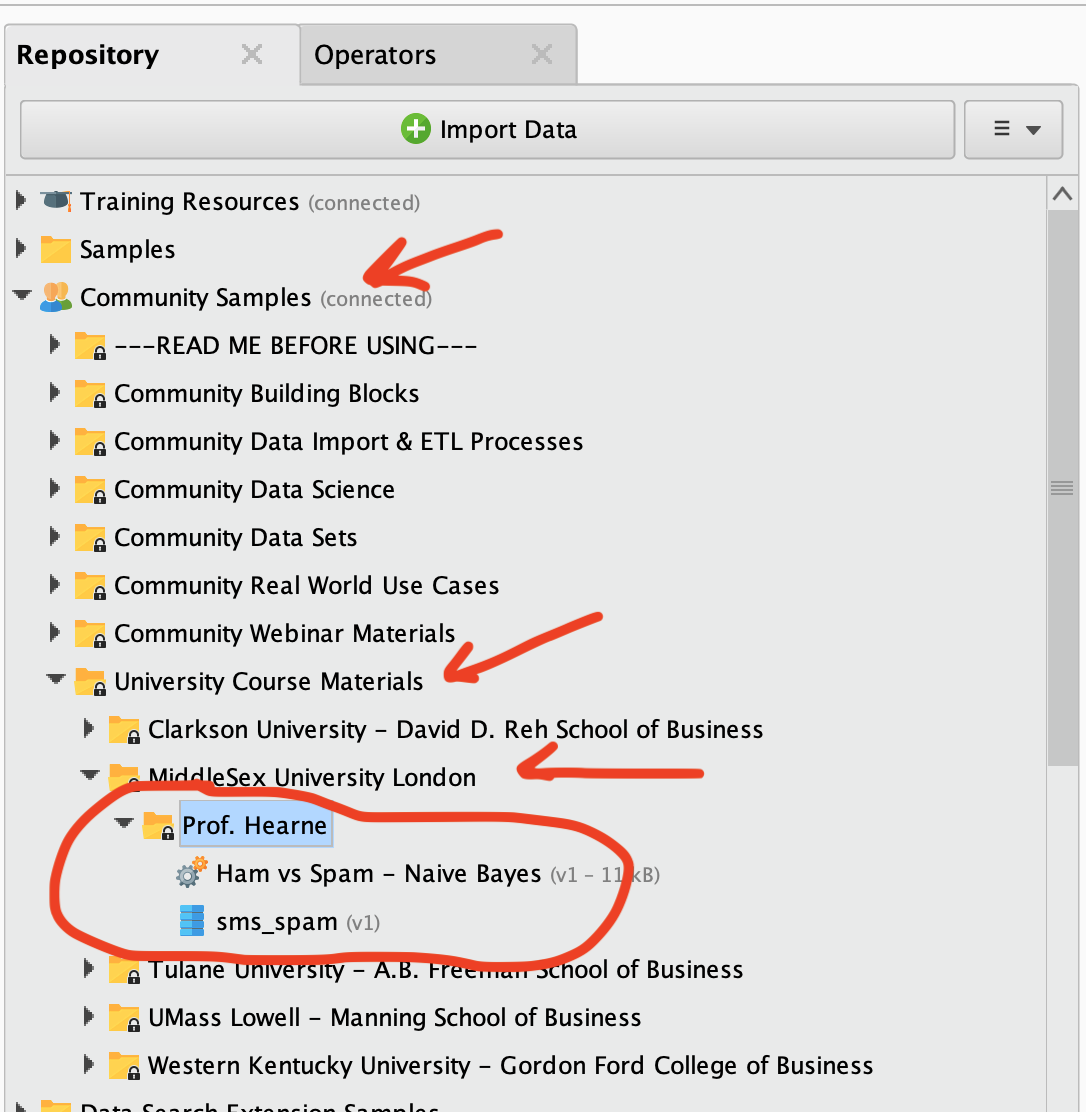Scott

• Administrator, Moderator, Employee, RapidMiner Certified Analyst, Community Manager, Member, University Professor, PM Moderator Posts: 2,959Community Manager
ok the grocery association rules one is also there: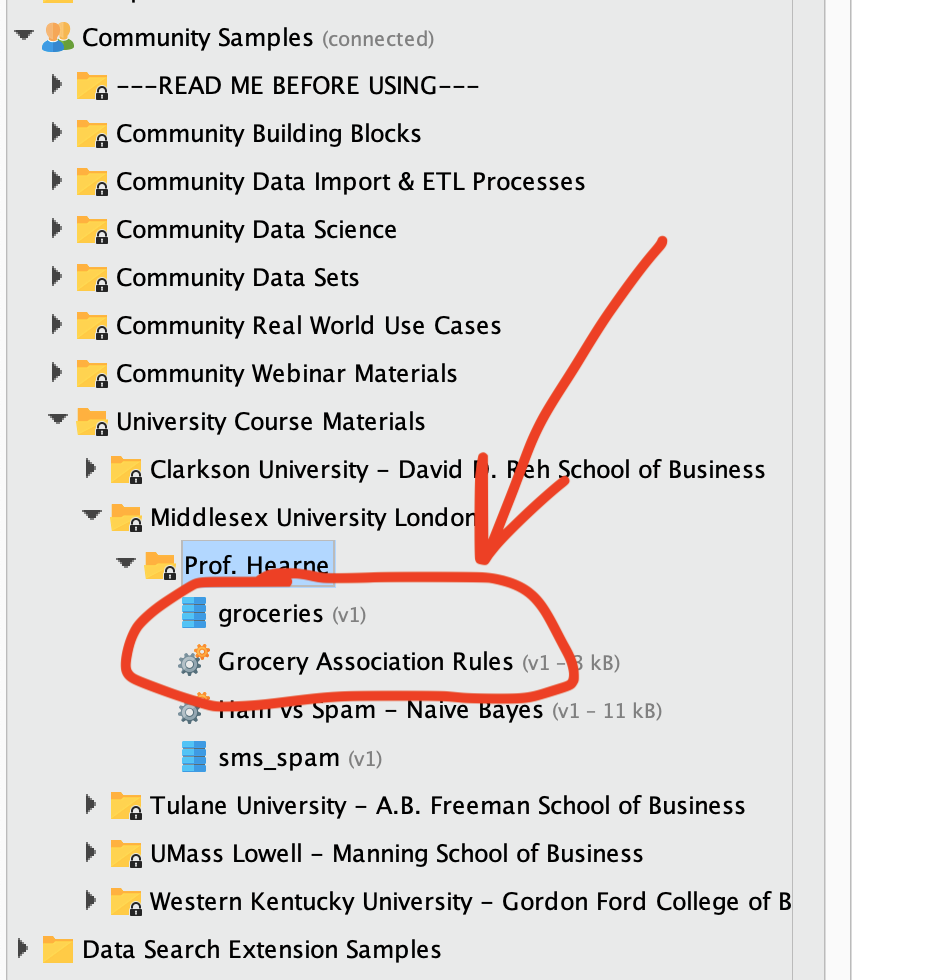Scott